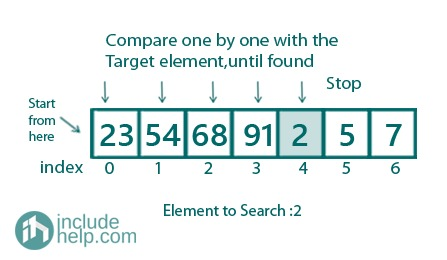# Python program for Linear Search

Linear search in Python: Here, we are going to learn to implement a linear search in an array or list in Python.
Submitted by Soumya Sinha, on December 28, 2020

Linear Search: Linear search is a searching algorithm which is used to search an element in an array or list.

Description:
Linear search is the traditional technique for searching an element in a collection of elements. In this sort of search, all the elements of the list are traversed one by one to search out if the element is present within the list or not.

Procedure for Linear Search:

```index = 0, flag = 0
For index is less than length(array)
If array[index] == number
flag = 1
Print element found at location (index +1) and
exit
If flag == 0
```

Example:

Consider a list <23, 54, 68, 91, 2, 5, 7>, suppose we are searching for element 2 in the list. Starting from the very first element we will compare each and every element of the list until we reach the index where 2 is present.Time Complexity: O(n)

## Python code for linear search

```import sys

def linear_search(arr, num_find):
# This function is used to search whether the given
# element is present within the list or not. If the element
# is present in list then the function will return its
# position in the list else it will return -1.
position = -1
for index in range(0, len(arr)):
if arr[index] == num_find:
position = index
break

return (position)

# main code
if __name__=='__main__':

arr = [10, 7, 2, 13, 4, 52, 6, 17, 81, 49]
num = 52
found = linear_search(arr, num)
if found != -1:
print('Number %d found at position %d'%(num, found+1))
else:
```

Output:

```Number 52 found at position 6
```

Preparation